# 数据结构|图

## 什么是"图"?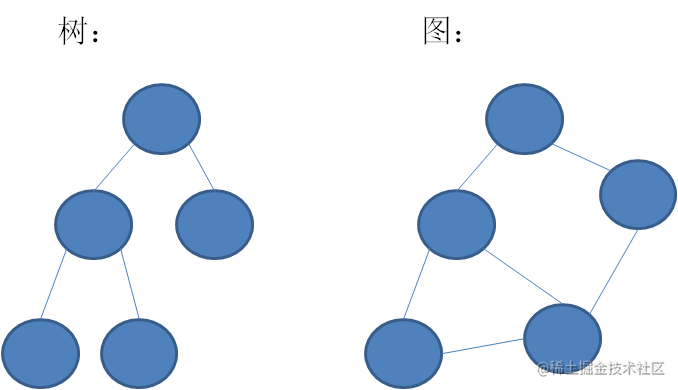## 图的相关术语

• V:一组顶点
• E:一组边，连接V中的顶点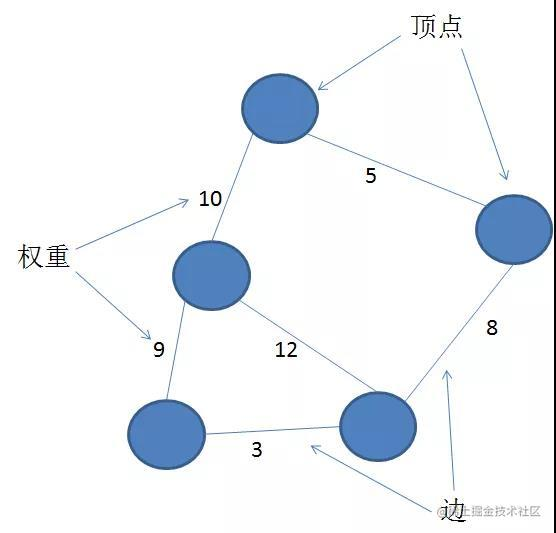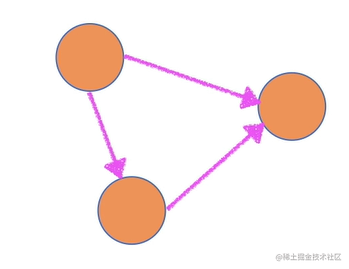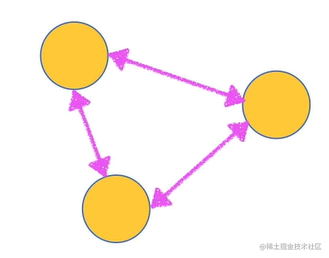## 常用代码实现图的几种方法

### 邻接矩阵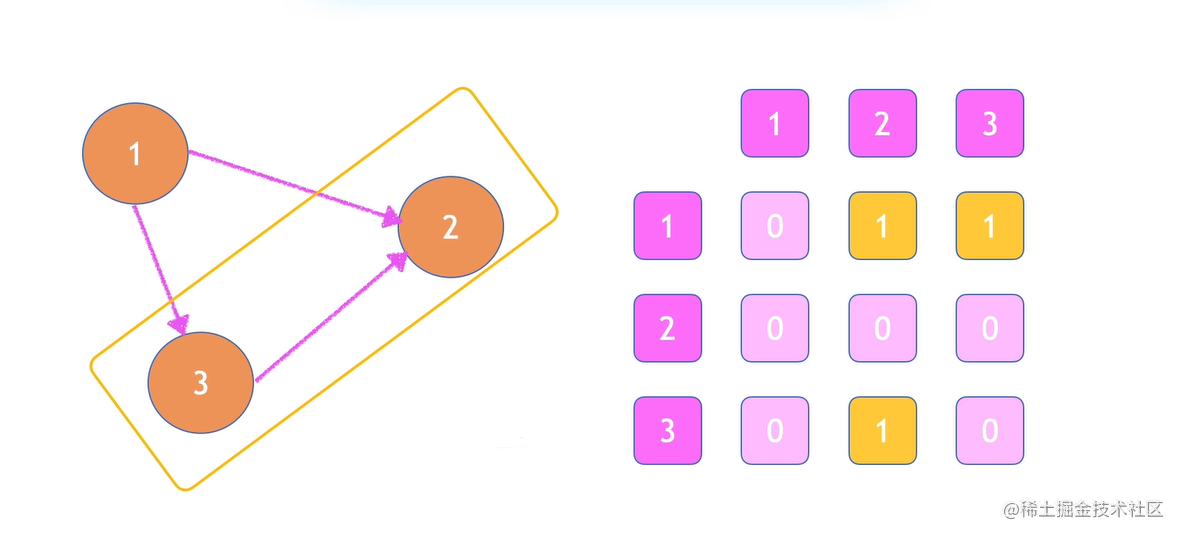``````let graphMatrix = [
[
0,1,1
],
[
0,0,0
],
[
0,1,0
]
]

### 邻接表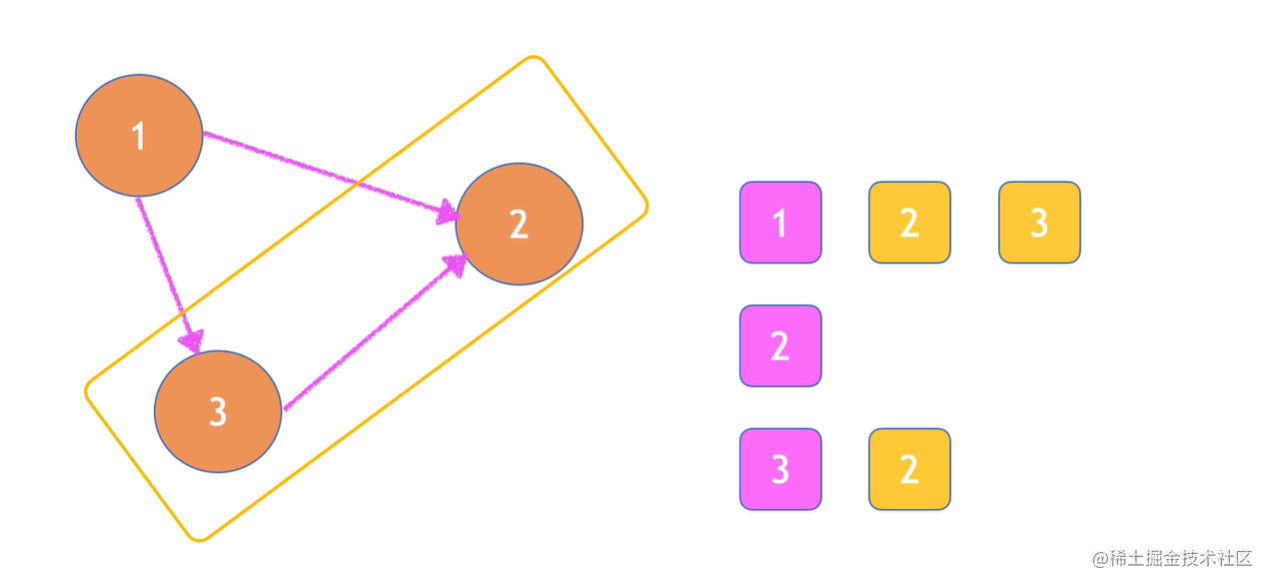## 代码实现

``````import Dictionary from "../Dictionary/app.js";

export default class Graph {
constructor(isDirected = false) {
this.isDirected = isDirected; // 接收isDirected表示图是否有向,默认情况下图是无向的
this.vertices = []; //初始化一个数组，用于存储图中所有顶点的名字
this.adjList = new Dictionary(); // 创建一个字典实例，用来存储邻接表(字典将会使用顶点的名字作为键-key，邻接顶点列表作为值-value)
}
/**
* 向图中添加一个新顶点
* @param {string} v 需要向图中添加的顶点
*/
if (!this.vertices.includes(v)) {
// 如果这个顶点不存在图中 Array.prototype.includes() 判断数组是否包含特定的元素
this.vertices.push(v); // 则将该顶点添加到顶点列表中
}
}
/**
* 向图中添加两点之间的边
* @param {string} a 传入要添加边的顶点a
* @param {string} b 传入要添加边的顶点b
*/
// 先校验两个顶点是否存在图中
//如果图中不存在顶点a，则将它加入顶点列表
}
// 如果图中不存在顶点b，则将它加入顶点列表
}
if (this.isDirected !== true) {
// 如果图为无向图
}
}
/**
* 返回图的顶点列表
* @returns {array}
*/
getVertices() {
return this.vertices;
}
/**
* 返回图的邻接表
* @returns {any}
*/
}
/**
* 将图以字符串的方式输出
* @returns {string}
*/
toString() {
let s = "";
for (let i = 0; i < this.vertices.length; i++) { // 迭代图的顶点列表(vertices)
s += `\${this.vertices[i]} -> `; // 将顶点的名字加入字符串中
const neighbors = this.adjList.get(this.vertices[i]); // 接着取得该顶点对应的邻接表
for (let j = 0; j < neighbors.length; j++) { //遍历该邻接表(neighbors)
s += `\${neighbors[j]} `; // 将相邻顶点加入我们的字符串
}
s += "\n"; // 邻接表迭代完成后，给我们的字符串添加一个换行符
}
return s; // 输入打印成字符串的图
/**
*  A -> B C D
B -> A E F
C -> A D G
D -> A C G H
E -> B I
F -> B
G -> C D
H -> D
I -> E
*/
}
}